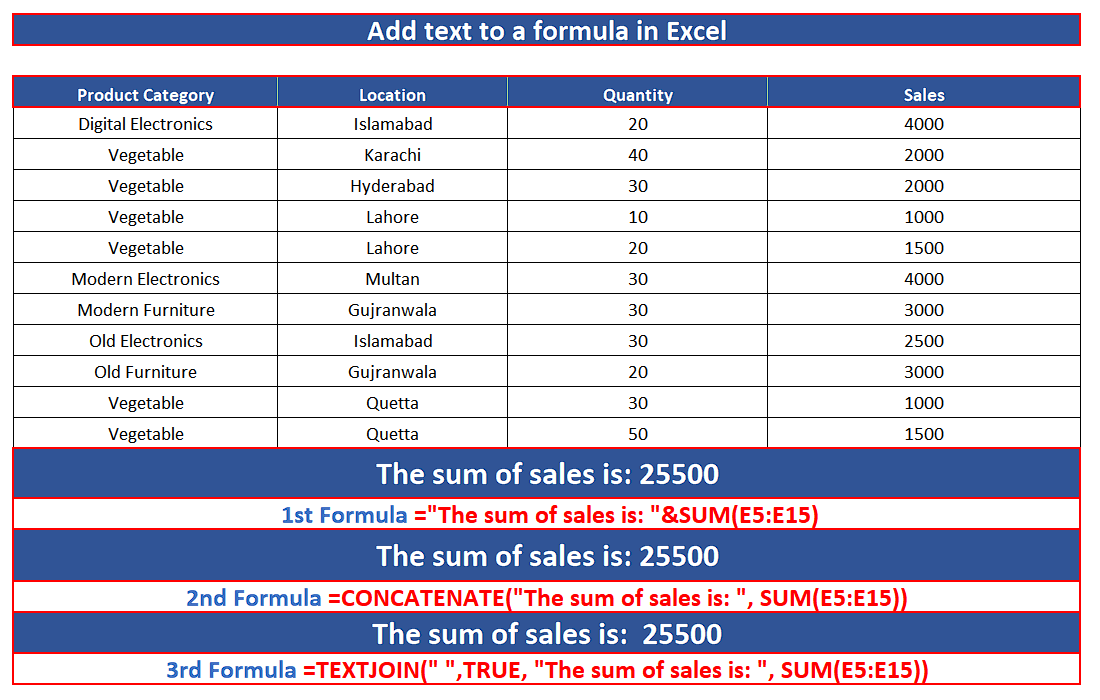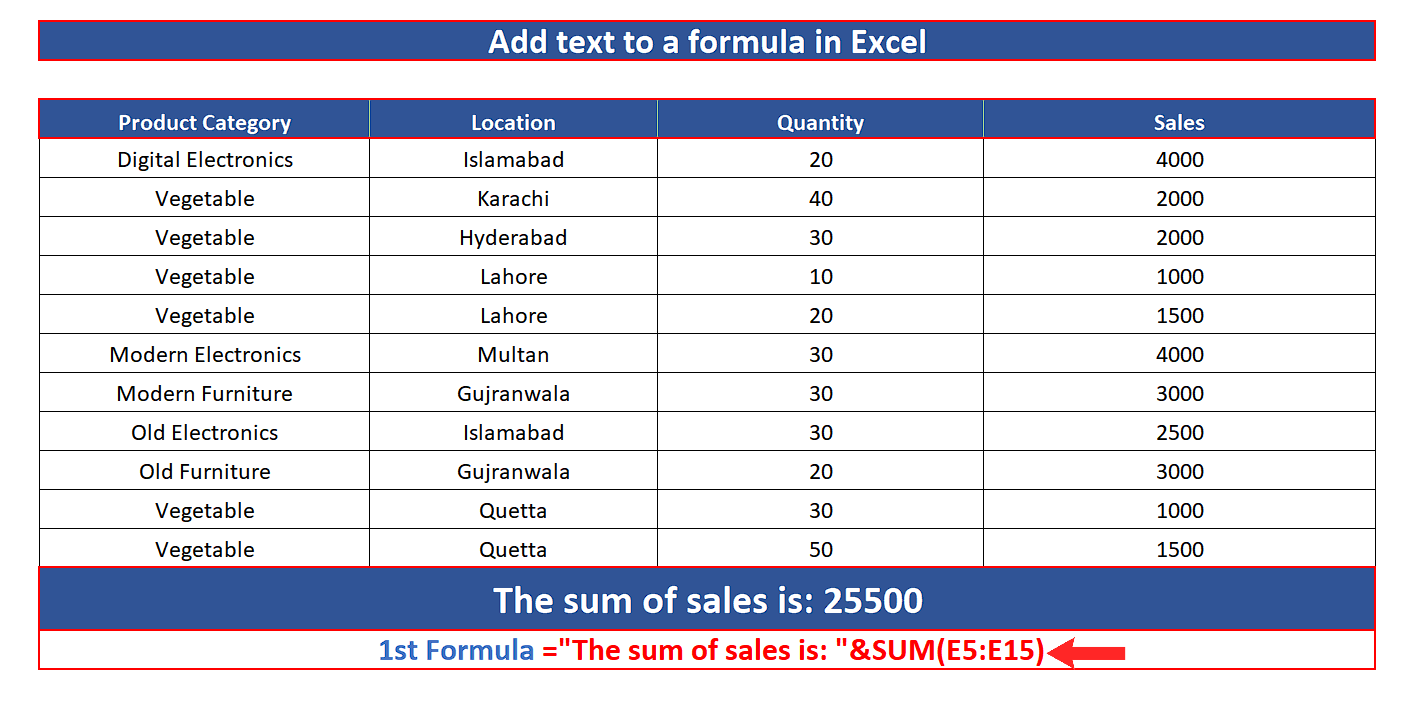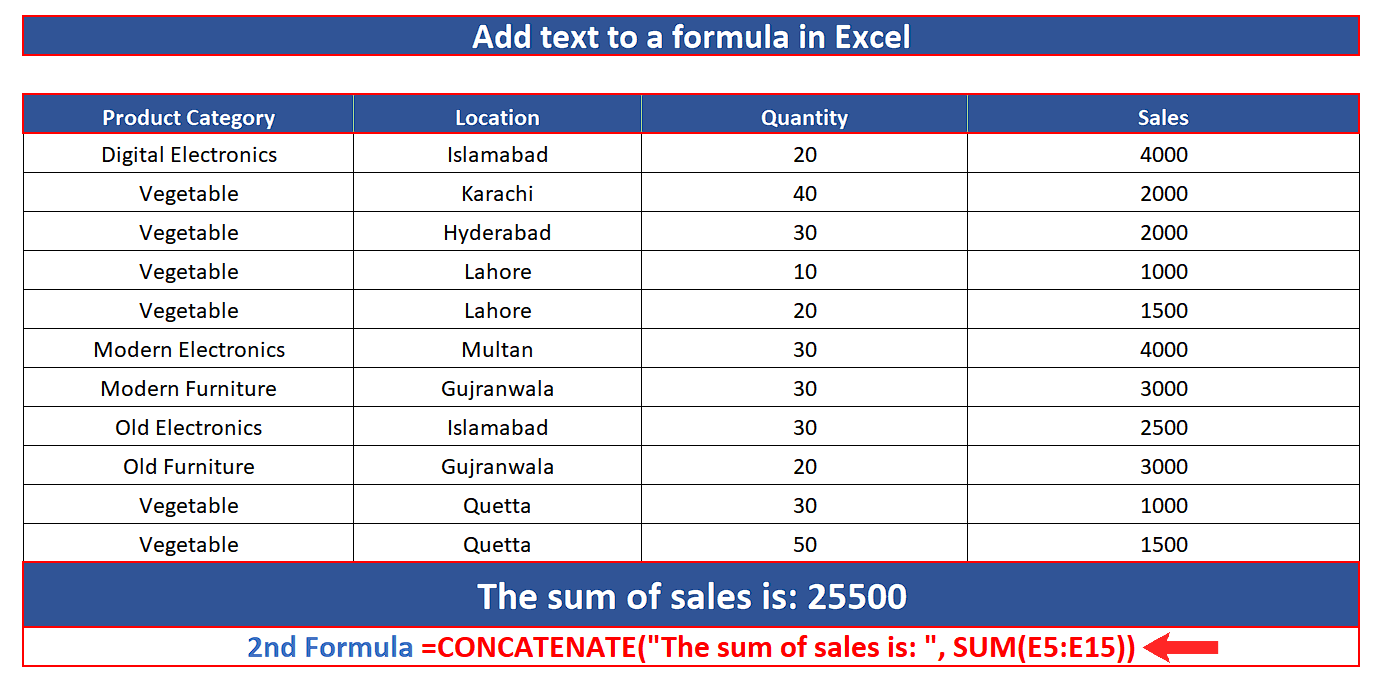# How to add text to a Formula in Excel

In today’s tutorial we’ll learn how to add a meaningful text to the results obtained by a formula in Excel. Let’s take a look at the following sales dataset.

We will calculate the sum of the sales for Vegetables by using SUMIF and then we’ll add some meaningful text to it by using & ampersand sign, CONCATENATE or TEXTJOIN.

Let’s follow the steps mentioned below and see how we can do it.Sometimes when you complete a complex calculation task or any data manipulation task in Excel and you are asked to add some text to the results obtained by a formula, to make the results more meaningful, then you certainly need to know how to add text to any results obtained by a formula in Excel.

### Step 1 – Add text to formula by using & Ampersand Sign– The simplest and easiest of all methods to add custom text to a formula is by using & Ampersand sign. Let’s assume that we have already calculated the total sum of the sales and we want to add the text “The sum of the sales is: ”  to the numeric result of our formula. For this purpose, write the following formula in any cell and press enter.
=”The sum of sales is: “&SUM(E5:E15)

The & ampersand sign simply joins any data that is mentioned before and after it. In our case we used two parameters,
The desired text “The sum of the sales is: ”  before & and the numeric result of SUM(E5:E15) formula after the & sign. The output is shown in the image above.

### Step 2 – Add text to formula by using CONCATENATE– The second method to add custom text to a formula is by using the CONCATENATE function. Let’s assume that we have already calculated the total sum of the sales and we want to add the text “The sum of the sales is: ”  to the numeric result of our formula. For this purpose, write the following formula in any cell and press enter.
=CONCATENATE(“The sum of sales is: “, SUM(E5:E15))

This function simply joins any data that is mentioned inside the function as parameters. In our case we used two parameters,
The desire text “The sum of the sales is: ”  and the numeric result of SUM(E5:E15) formula. The output is shown in the image above.

### Step 3 – Add text to formula by using TEXTJOIN– The third method to add custom text to a formula is by using the TEXTJOIN function. Let’s assume that we have already calculated the total sum of the sales and we want to add the text “The sum of the sales is: ”  to the numeric result of our formula. For this purpose, write the following formula in any cell and press enter.
=TEXTJOIN(“ ”, “The sum of sales is: “, SUM(E5:E15))

This function simply joins any data that is mentioned inside the function as parameters, separated by a specific delimiter which is the first parameter of this function. In our case we used three parameters,
“ ” blank space as delimiter to add a space between text and numeric data, Our Text “The sum of the sales is: ”  and the numeric result of SUM(E5:E15) formula. The output of the formula is shown in the image above.